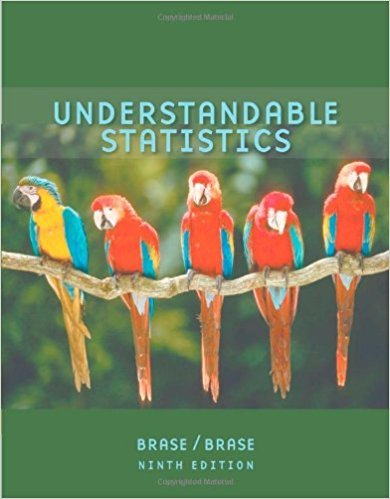×
×

# Solutions for Chapter 9.5: ESTIMATION## Full solutions for Understandable Statistics | 9th Edition

ISBN: 9780618949922Solutions for Chapter 9.5: ESTIMATION

Solutions for Chapter 9.5
4 5 0 289 Reviews
30
3
##### ISBN: 9780618949922

This expansive textbook survival guide covers the following chapters and their solutions. This textbook survival guide was created for the textbook: Understandable Statistics, edition: 9. Since 32 problems in chapter 9.5: ESTIMATION have been answered, more than 33696 students have viewed full step-by-step solutions from this chapter. Chapter 9.5: ESTIMATION includes 32 full step-by-step solutions. Understandable Statistics was written by and is associated to the ISBN: 9780618949922.

Key Statistics Terms and definitions covered in this textbook
• Additivity property of x 2

If two independent random variables X1 and X2 are distributed as chi-square with v1 and v2 degrees of freedom, respectively, Y = + X X 1 2 is a chi-square random variable with u = + v v 1 2 degrees of freedom. This generalizes to any number of independent chi-square random variables.

A variation of the R 2 statistic that compensates for the number of parameters in a regression model. Essentially, the adjustment is a penalty for increasing the number of parameters in the model. Alias. In a fractional factorial experiment when certain factor effects cannot be estimated uniquely, they are said to be aliased.

• Alias

In a fractional factorial experiment when certain factor effects cannot be estimated uniquely, they are said to be aliased.

• Analytic study

A study in which a sample from a population is used to make inference to a future population. Stability needs to be assumed. See Enumerative study

• Bias

An effect that systematically distorts a statistical result or estimate, preventing it from representing the true quantity of interest.

• Combination.

A subset selected without replacement from a set used to determine the number of outcomes in events and sample spaces.

• Continuity correction.

A correction factor used to improve the approximation to binomial probabilities from a normal distribution.

• Correlation

In the most general usage, a measure of the interdependence among data. The concept may include more than two variables. The term is most commonly used in a narrow sense to express the relationship between quantitative variables or ranks.

• Correlation matrix

A square matrix that contains the correlations among a set of random variables, say, XX X 1 2 k , ,…, . The main diagonal elements of the matrix are unity and the off-diagonal elements rij are the correlations between Xi and Xj .

• Covariance matrix

A square matrix that contains the variances and covariances among a set of random variables, say, X1 , X X 2 k , , … . The main diagonal elements of the matrix are the variances of the random variables and the off-diagonal elements are the covariances between Xi and Xj . Also called the variance-covariance matrix. When the random variables are standardized to have unit variances, the covariance matrix becomes the correlation matrix.

• Crossed factors

Another name for factors that are arranged in a factorial experiment.

• Curvilinear regression

An expression sometimes used for nonlinear regression models or polynomial regression models.

• Designed experiment

An experiment in which the tests are planned in advance and the plans usually incorporate statistical models. See Experiment

• Discrete distribution

A probability distribution for a discrete random variable

• Discrete random variable

A random variable with a inite (or countably ininite) range.

• Distribution free method(s)

Any method of inference (hypothesis testing or conidence interval construction) that does not depend on the form of the underlying distribution of the observations. Sometimes called nonparametric method(s).

• Empirical model

A model to relate a response to one or more regressors or factors that is developed from data obtained from the system.

• Enumerative study

A study in which a sample from a population is used to make inference to the population. See Analytic study

• Erlang random variable

A continuous random variable that is the sum of a ixed number of independent, exponential random variables.

• Error mean square

The error sum of squares divided by its number of degrees of freedom.

×